您可以捐助，支持我们的公益事业。 1元 10元 50元 认证码：必填求知 文章 文库 Lib 视频 iPerson 课程 认证 咨询 工具 讲座 Modeler Code要资料订阅捐助

 176 次浏览     评价： 好 中 差
2020-7-14

 编辑推荐: 本文使用OpenCV简介，Opencv的安装、图像颜色模型的转换与图形绘制等相关内容，详情请看下文。 本文来自于云+社区，由火龙果软件Anna编辑、推荐。

1. 理解颜色模型与在图像上绘制图形（图像处理基本操作）。

2. 基本的图像处理与过滤。

3. 从特征检测到人脸检测（TBU）

OpenCV是Open Source Computer Vision的缩写，由英特尔公司于1999年推出。它最初是用C/ C++编写的，所以你可能会看到更多用C语言而不是Python编写的教程。但现在它在Python中也被广泛用于计算机视觉。首先，让我们为使用OpenCV配置环境。安装过程如下，详细安装描述参看（https://pypi.org/project/opencv-python/）。

 pip install opencv-python==3.4.2 pip install opencv-contrib-python==3.3.1

 import cv2 cv2.__version__

 import numpy as np import matplotlib.pyplot as plt %matplotlib inline # Import the image img = cv2.imread('burano.jpg') plt.imshow(img)# Convert the image into RGB img_rgb = cv2.cvtColor(img, cv2.COLOR_BGR2RGB) plt.imshow(img_rgb)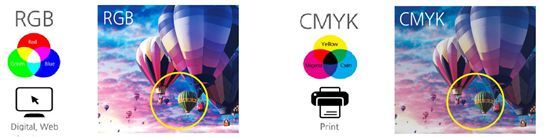# Convert the image into gray scale img_gray = cv2.cvtColor(img, cv2.COLOR_BGR2GRAY) plt.imshow(img_gray, cmap = 'gray')

 # Plot the three channels of the image fig, axs = plt.subplots(nrows = 1, ncols = 3,figsize = (20, 20)) for i in range(0, 3): ax =axs[i] ax.imshow(img_rgb[:, :, i], cmap = 'gray')plt.show()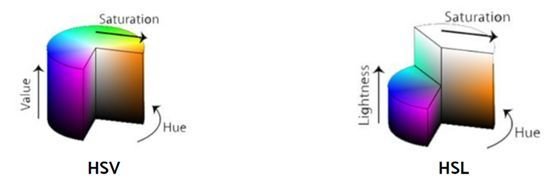HSV和HLS有一些不同。正如在上图看到的那样，他们有一个三维的表达，更类似于人类的感知方式。HSV代表色调、饱和度和色值。HSL代表色调、饱和度和亮度。HSV的中轴是色值，HSL的中轴是光量。沿着中心轴的角度，有色调和实际的颜色。与中心轴的距离属于饱和度。转换颜色模型的方法如下。

 # Transform the image into HSV and HLS models img_hsv = cv2.cvtColor(img, cv2.COLOR_BGR2HSV) img_hls = cv2.cvtColor(img, cv2.COLOR_BGR2HLS) # Plot the converted images fig, (ax1, ax2) = plt.subplots(nrows = 1, ncols =2, figsize = (20, 20)) ax1.imshow(img_hsv) ax2.imshow(img_hls) plt.show()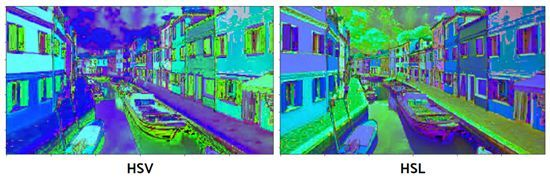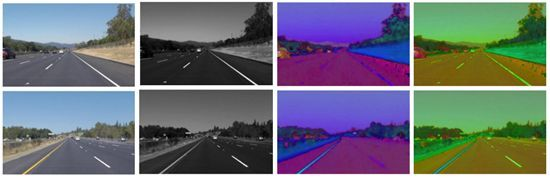# Copy the image img_copy = img.copy() # Draw a rectangle cv2.rectangle(img_copy, pt1 = (800, 470), pt2 =(980, 530), color = (255, 0, 0), thickness = 5) plt.imshow(img_copy)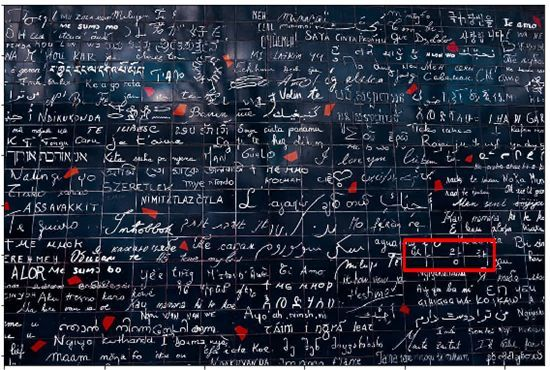# Draw a circle cv2.circle(img_copy, center = (950, 50), radius =50, color = (0, 0, 255), thickness = 5) plt.imshow(img_copy)，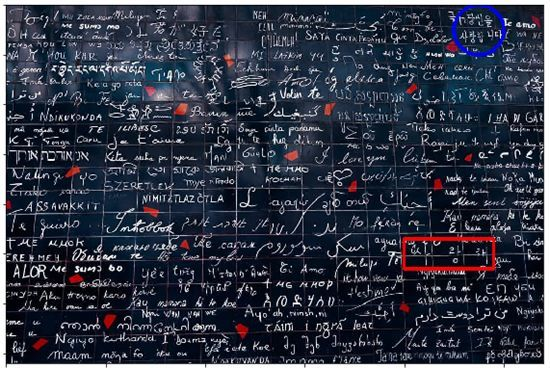# Add text cv2.putText(img_copy, text = "the Wall ofLove", org = (250, 250), fontFace = cv2.FONT_HERSHEY_DUPLEX, fontScale = 2, color = (0, 255, 0), thickness = 2, lineType = cv2.LINE_AA) plt.imshow(img_copy)

 # Step 1. Define callback function def draw_circle(event, x, y, flags, param): ifevent == cv2.EVENT_LBUTTONDOWN: cv2.circle(img, center = (x, y), radius = 5, color = (87, 184, 237), thickness = -1) elifevent == cv2.EVENT_RBUTTONDOWN: cv2.circle(img, center = (x, y), radius = 10, color = (87, 184, 237),thickness = 1)

cv2.EVENT_RBUTTONDOWN记录位置数据。把鼠标的位置设置为圆心(x, y)，并绘制圆圈。

 # Step 2. Call the window img = cv2.imread('map.png') cv2.namedWindow(winname = 'my_drawing') cv2.setMouseCallback('my_drawing', draw_circle)

 # Step 3. Execution while True: cv2.imshow('my_drawing',img) ifcv2.waitKey(10) & 0xFF == 27: break cv2.destroyAllWindows()# Initialization drawing = False ix = -1 iy = -1 # create a drawing function def draw_rectangle(event, x, y, flags, params): globalix, iy, drawing ifevent == cv2.EVENT_LBUTTONDOWN: drawing = True ix,iy = x, y elifevent == cv2.EVENT_MOUSEMOVE: ifdrawing == True: cv2.rectangle(img, pt1=(ix, iy), pt2=(x, y), color = (87, 184,237), thickness = -1) elifevent == cv2.EVENT_LBUTTONUP: drawing = False cv2.rectangle(img, pt1=(ix, iy), pt2=(x, y), color = (87, 184, 237),thickness = -1)

 import cv2 import numpy as np# Step 1. Define callback function drawing = False ix = -1 iy = -1 def draw_rectangle(event, x, y, flags, params): globalix, iy, drawing ifevent == cv2.EVENT_LBUTTONDOWN: drawing = True ix,iy = x, y elifevent == cv2.EVENT_MOUSEMOVE: ifdrawing == True: cv2.rectangle(img, pt1 = (ix, iy), pt2 = (x, y), color = (87, 184,237), thickness = -1) elifevent == cv2.EVENT_LBUTTONUP: drawing = False cv2.rectangle(img, pt1 = (ix, iy), pt2 = (x, y), color = (87, 184, 237),thickness = -1) # Step 2. Call the window img = cv2.imread('map.png')cv2.namedWindow(winname = 'my_drawing') cv2.setMouseCallback('my_drawing',draw_rectangle)# Step 3. Execution while True: cv2.imshow('my_drawing', img) ifcv2.waitKey(10) & 0xFF == 27: breakcv2.destroyAllWindows()

 176 次浏览     评价： 好 中 差订阅捐助
 相关文章 我们该如何设计数据库 数据库设计经验谈 数据库设计过程 数据库编程总结
 相关文档 数据库性能调优技巧 数据库性能调整 数据库性能优化讲座 数据库系统性能调优系列讲座 前端开发框架uni-app 主讲：苏雯斐 时间：2020年8月15日实录 企业架构师的能力模型 主讲：俎涛